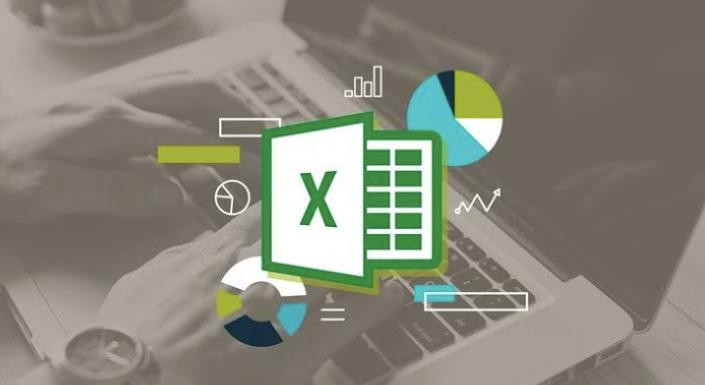G-8XHNS3HXSS

•SkillEnable

# The Ultimate Guide on ExcelSince its launch in 1985, Microsoft Excel has not only become the leading name in spreadsheet software but arguably also a second home (and language) to accountants and CFOs alike.

To begin with a simple question:

## What is excel?

Microsoft Excel is a helpful and powerful program for data analysis and documentation. It is a spreadsheet program, which contains a number of columns and rows, where each intersection of a column and a row is a “cell.” Each cell contains one point of data or one piece of information. By organizing the information in this way, you can make information easier to find, and automatically draw information from changing data.## Features of Excel:

MS Excel allows us to keep the header and footer in our spreadsheet document.

2. Find and Replace Command

MS Excel allows us to find the needed data (text and numbers) in the workbook and also replace the existing data with a new one.

It allows the user to protect their workbooks by using a password from unauthorized access to their information.

4. Data Filtering

Filtering is a quick and easy way to find and work with a subset of data in a range. A filtered range displays only the rows that meet the criteria you specify for a column. MS Excel provides two commands for filtering ranges:

• Auto Filter; which includes filter by selection, for simple criteria

• Advanced Filter; for more complex criteria.

5. Data Sorting

Data sorting is the process of arranging data in some logical order. MS Excel allows us to sort data either in ascending or descending order.

6. Built-in formulae

MS Excel has got many built-in formulae for sum, average, minimum, etc. We can use those formulae as per our needs.

7. Create different charts

(Pivot Table Report)

MS Excel allows us to create different charts such as bar graphs, pie- charts, line graphs, etc. This helps us to analyze and compare data very easily.

8. Automatically edits the result

MS Excel automatically edits the result if any changes are made in any of the cells.

9. Formula Auditing

Using formula auditing we can graphically display or trace the relationships between cells and formulas with blue arrows. We can trace the precedents (the cells that provide data to a specific cell) or the dependents (the cells that depend on the value).

## Basic formulas for a smooth workflow.

1.SUM

The SUM function is the first must-know formula in Excel. It usually aggregates values from a selection of columns or rows from your selected range.

=SUM(number1, [number2], …)

2. AVERAGE

The AVERAGE function should remind you of simple averages of data such as the average number of shareholders in a given shareholding pool.

=AVERAGE(number1, [number2], …)

3.COUNT

The COUNT function counts all cells in a given range that contain only numeric values.

=COUNT(value1, [value2], …)

4. IF

The IF function is often used when you want to sort your data according to a given logic. The best part of the IF formula is that you can embed formulas and function in it.

=IF(logical_test, [value_if_true], [value_if_false])

5. MAX & MIN

The MAX and MIN functions help in finding the maximum number and the minimum number in a range of values.

=MIN(number1, [number2], …)### Basic Functions:

The main uses of Excel include:

● Data entry

● Data management

● Accounting

● Financial analysis

● Charting and graphing

● Programming

● Time management

● Financial modeling

● Customer relationship management (CRM)

Almost anything that needs to be organized!

Visit www.skillenable.com today!

Source: Microsoft EXCEL BIBLE- The comprehensive tutorial resource.